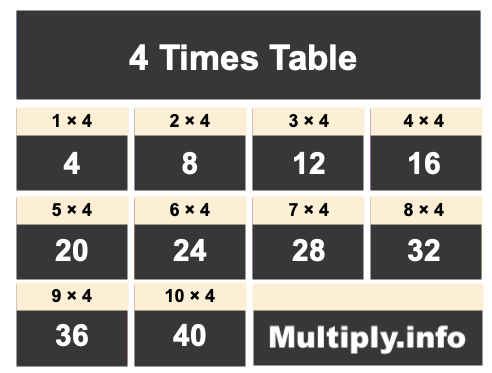4 Times TableThe picture above shows the first ten numbers in the 4 Times Table. It is created my multiplying 4 by one, then by two, then by three, and so on all the way up to ten.

Students and others use our 4 Times Table for many things, such as to find patterns or for memorizing products of numbers multiplied by 4.

In addition to the image of the 4 Times Table above, we have also written the 4 Times Table below showing 4 multiplied by every number up to one hundred. The list is in ascending order.

We created the 4 Times Table below to make it easy to read and print. We hope you will find our 4 Times Table helpful.

4 x  0 = 0
4 x  1 = 4
4 x  2 = 8
4 x  3 = 12
4 x  4 = 16
4 x  5 = 20
4 x  6 = 24
4 x  7 = 28
4 x  8 = 32
4 x  9 = 36
4 x 10 = 40
4 x 11 = 44
4 x 12 = 48
4 x 13 = 52
4 x 14 = 56
4 x 15 = 60
4 x 16 = 64
4 x 17 = 68
4 x 18 = 72
4 x 19 = 76
4 x 20 = 80
4 x 21 = 84
4 x 22 = 88
4 x 23 = 92
4 x 24 = 96
4 x 25 = 100
4 x 26 = 104
4 x 27 = 108
4 x 28 = 112
4 x 29 = 116
4 x 30 = 120
4 x 31 = 124
4 x 32 = 128
4 x 33 = 132
4 x 34 = 136
4 x 35 = 140
4 x 36 = 144
4 x 37 = 148
4 x 38 = 152
4 x 39 = 156
4 x 40 = 160
4 x 41 = 164
4 x 42 = 168
4 x 43 = 172
4 x 44 = 176
4 x 45 = 180
4 x 46 = 184
4 x 47 = 188
4 x 48 = 192
4 x 49 = 196
4 x 50 = 200
4 x 51 = 204
4 x 52 = 208
4 x 53 = 212
4 x 54 = 216
4 x 55 = 220
4 x 56 = 224
4 x 57 = 228
4 x 58 = 232
4 x 59 = 236
4 x 60 = 240
4 x 61 = 244
4 x 62 = 248
4 x 63 = 252
4 x 64 = 256
4 x 65 = 260
4 x 66 = 264
4 x 67 = 268
4 x 68 = 272
4 x 69 = 276
4 x 70 = 280
4 x 71 = 284
4 x 72 = 288
4 x 73 = 292
4 x 74 = 296
4 x 75 = 300
4 x 76 = 304
4 x 77 = 308
4 x 78 = 312
4 x 79 = 316
4 x 80 = 320
4 x 81 = 324
4 x 82 = 328
4 x 83 = 332
4 x 84 = 336
4 x 85 = 340
4 x 86 = 344
4 x 87 = 348
4 x 88 = 352
4 x 89 = 356
4 x 90 = 360
4 x 91 = 364
4 x 92 = 368
4 x 93 = 372
4 x 94 = 376
4 x 95 = 380
4 x 96 = 384
4 x 97 = 388
4 x 98 = 392
4 x 99 = 396
4 x 100 = 400

Times Table Charts
Enter another number below to see the Times Table for that number.

5 Times Table
Did you find useful patterns in our 4 Times Table that you can use for the future? Check out the next Times Table on our list.